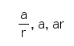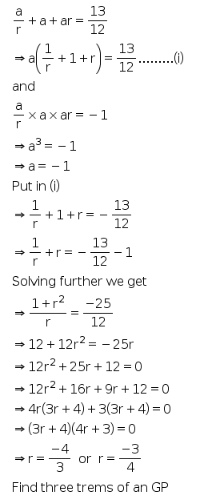# The sum of the first three terms of a G.p is 13/12 and their product is -1

The sum of the first three terms of a G.p is 13/12 and their product is -1. Find the G.p?

Let terms of the geometric progression are